Publicité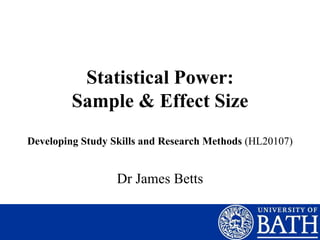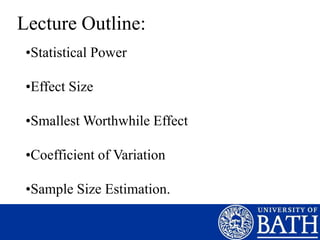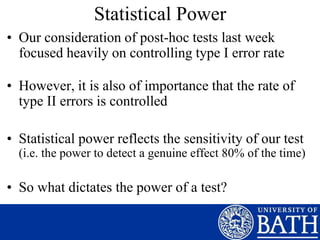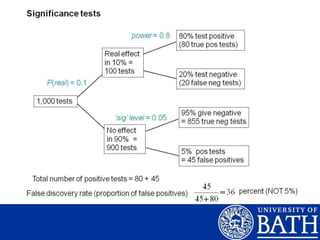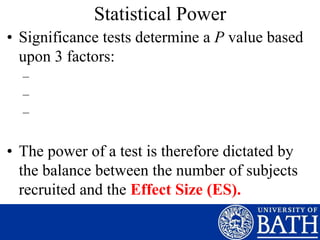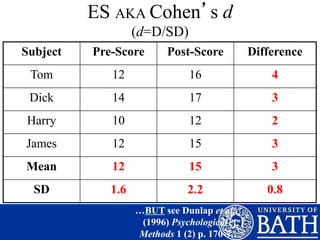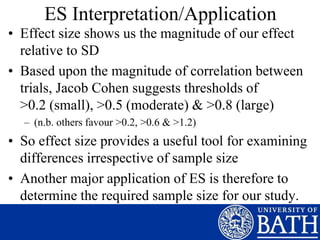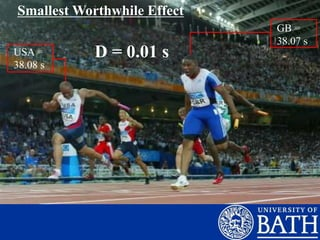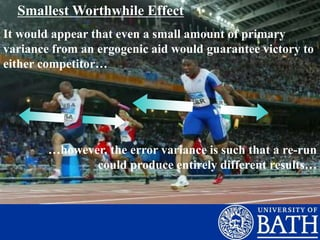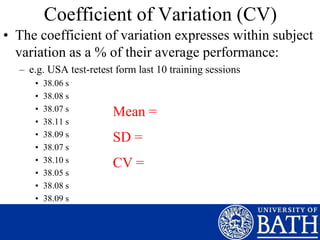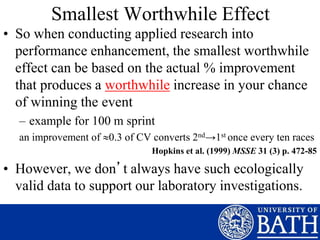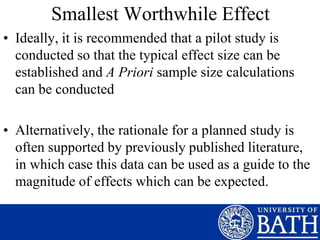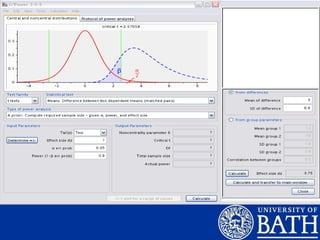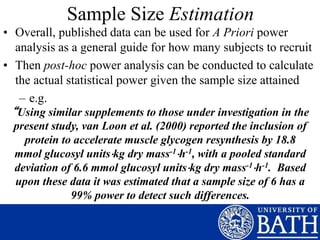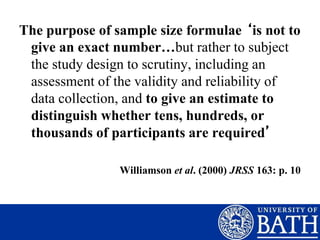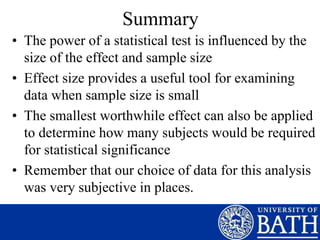1 sur 17
Publicité

### Lecture 5 Statistical Power (Handout).ppt

1. Statistical Power: Sample & Effect Size Dr James Betts Developing Study Skills and Research Methods (HL20107)
2. Lecture Outline: •Statistical Power •Effect Size •Smallest Worthwhile Effect •Coefficient of Variation •Sample Size Estimation.
3. Statistical Power • Our consideration of post-hoc tests last week focused heavily on controlling type I error rate • However, it is also of importance that the rate of type II errors is controlled • Statistical power reflects the sensitivity of our test (i.e. the power to detect a genuine effect 80% of the time) • So what dictates the power of a test?
4. Statistical Power • Significance tests determine a P value based upon 3 factors: – – – • The power of a test is therefore dictated by the balance between the number of subjects recruited and the Effect Size (ES).
5. ES AKA Cohen’s d (d=D/SD) Subject Pre-Score Post-Score Difference Tom 12 16 4 Dick 14 17 3 Harry 10 12 2 James 12 15 3 Mean 12 15 3 SD 1.6 2.2 0.8 …BUT see Dunlap et al. (1996) Psychological Methods 1 (2) p. 170-7
6. ES Interpretation/Application • Effect size shows us the magnitude of our effect relative to SD • Based upon the magnitude of correlation between trials, Jacob Cohen suggests thresholds of >0.2 (small), >0.5 (moderate) & >0.8 (large) – (n.b. others favour >0.2, >0.6 & >1.2) • So effect size provides a useful tool for examining differences irrespective of sample size • Another major application of ES is therefore to determine the required sample size for our study.
7. GB = 38.07 s USA = 38.08 s Smallest Worthwhile Effect D = 0.01 s
8. Smallest Worthwhile Effect It would appear that even a small amount of primary variance from an ergogenic aid would guarantee victory to either competitor… …however, the error variance is such that a re-run could produce entirely different results…
9. …for an effect to guarantee first place, it would need to exceed the opponents time by more than his error variance. Re-Run
10. Coefficient of Variation (CV) • The coefficient of variation expresses within subject variation as a % of their average performance: – e.g. USA test-retest form last 10 training sessions • 38.06 s • 38.08 s • 38.07 s • 38.11 s • 38.09 s • 38.07 s • 38.10 s • 38.05 s • 38.08 s • 38.09 s Mean = SD = CV =
11. Smallest Worthwhile Effect • So when conducting applied research into performance enhancement, the smallest worthwhile effect can be based on the actual % improvement that produces a worthwhile increase in your chance of winning the event – example for 100 m sprint an improvement of 0.3 of CV converts 2nd→1st once every ten races Hopkins et al. (1999) MSSE 31 (3) p. 472-85 • However, we don’t always have such ecologically valid data to support our laboratory investigations.
12. Smallest Worthwhile Effect • Ideally, it is recommended that a pilot study is conducted so that the typical effect size can be established and A Priori sample size calculations can be conducted • Alternatively, the rationale for a planned study is often supported by previously published literature, in which case this data can be used as a guide to the magnitude of effects which can be expected.
13. Sample Size Estimation • Overall, published data can be used for A Priori power analysis as a general guide for how many subjects to recruit • Then post-hoc power analysis can be conducted to calculate the actual statistical power given the sample size attained – e.g. “Using similar supplements to those under investigation in the present study, van Loon et al. (2000) reported the inclusion of protein to accelerate muscle glycogen resynthesis by 18.8 mmol glucosyl unitskg dry mass-1h-1, with a pooled standard deviation of 6.6 mmol glucosyl unitskg dry mass-1h-1. Based upon these data it was estimated that a sample size of 6 has a 99% power to detect such differences.
14. The purpose of sample size formulae ‘is not to give an exact number…but rather to subject the study design to scrutiny, including an assessment of the validity and reliability of data collection, and to give an estimate to distinguish whether tens, hundreds, or thousands of participants are required’ Williamson et al. (2000) JRSS 163: p. 10
15. Summary • The power of a statistical test is influenced by the size of the effect and sample size • Effect size provides a useful tool for examining data when sample size is small • The smallest worthwhile effect can also be applied to determine how many subjects would be required for statistical significance • Remember that our choice of data for this analysis was very subjective in places.
Publicité